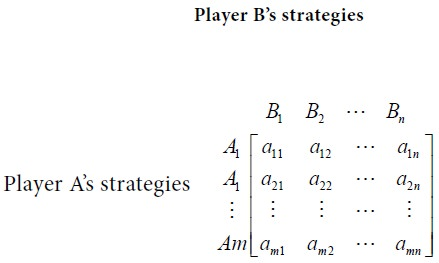Home | ARTS | Operations Management | Definition of two-person zero sum game

# Definition of two-person zero sum game

Posted On :  24.06.2018 09:47 pm

A game with only two players, say player A and player B, is called a two-person zero sum game if the gain of the player A is equal to the loss of the player B, so that the total sum is zero.

Definition of two-person zero sum game

A game with only two players, say player A and player B, is called a two-person zero sum game if the gain of the player A is equal to the loss of the player B, so that the total sum is zero.

## Payoff matrix

When players select their particular strategies, the payoffs (gains or losses) can be represented in the form of a payoff matrix.

Since the game is zero sum, the gain of one player is equal to the loss of other and vice-versa. Suppose A has m strategies and B has n strategies. Consider the following payoff matrix.Player A wishes to gain as large a payoff aij as possible while player B will do his best to reach as small a value aij as possible where the gain to player B and loss to player A be (- aij ).

Tags : Operations Management - Game Theory, Goal Programming & Queuing Theory
Last 30 days 549 views